# Self-Healing Performance of Rubber-Modified Asphalt

Self-Healing Performance of Rubber-Modified Asphalt
School of Construction Engineering, Jilin University, Changchun 130026, China

Corresponding Author Email:
luoyang19@mails.jlu.edu.cn
Page:
75-80
|
DOI:
https://doi.org/10.18280/eesrj.080202
10 March 2021
|
Accepted:
25 May 2021
|
Published:
30 June 2021
| Citation

OPEN ACCESS

Abstract:

To figure out the effect of rubber modifiers on the self-healing performance of asphalt, this study took Eucommia rubber (TPI, trans-polyisoprene) and natural rubber (NR) as asphalt modifiers and employed the molecule simulation software to analyze the self-healing performance of asphalt in the microscale. At first, a model of basic asphalt system was established in the molecule simulation software, and three parameters of density, solubility and mean square displacement (MSD) were used to verify that the established model can act as the representative of asphalt molecules; then, several self-healing models of basic asphalt and rubber-modified asphalt were constructed to compare the properties of TPI and NR. In the experiment, the temperature was set to 303.15K, 313.15K, 323.15K, 333.15K, and 343.15K, respectively, and a 10Å (1Å=0.1nm) vacuum layer was taken as the microcrack of the asphalt, then the entire self-healing process of the models was analyzed, and the diffusion coefficient and energy change of the models were calculated. The experimental results revealed that, the NR-modified asphalt outperformed TPI in self-healing performance; for the NR-modified asphalt, as the temperature rises, the intermolecular non-bond energy changes, the molecule diffusion ability enhances, which can accelerate the self-healing progress of the microcracks in the asphalt.

Keywords:

road engineering, self-healing, modified asphalt, molecule simulation, rubber asphalt, molecular dynamics

1. Introduction

With the progress of industrialization in China, the demand for rubber is increasing significantly, and NR is in short supply, in this context, TPI is often used as a substitute of NR. TPI is a type of natural high molecular polymer, and its main chemical composition is trans-1,4-polyisoprene, which is the same as NR (cis-1,4-polyisoprene), and the two are isomers . Scholar Xu et al.  used molecular dynamics to compare pure TPI and TPI/NR blends and proved that the blends have better static mechanical properties and dynamic tensile fatigue resistance. Zhang et al.  used molecular dynamics to analyze the glass transition temperature of TPI and NR and verified the results through DSC experiment. Wang  believes that elastic recovery and the existence of hydrogen bond networks are the main mechanisms for the self-repair of rubber system. Xuan et al.  analyzed the self-healing performance of rubber asphalt based on viscoelastic theory, and adopted temperature difference displayer and time scanner to compare the size of the stress and the impact of damage degree on the self-healing performance. This study employed the Materials studio software to study and compare the self-healing performance of NR-modified asphalt, TPI-modified asphalt, and basic asphalt in the microscale.

2. Construction and Verification of Asphalt Model

2.1 Modeling

Asphalt has a very complex molecular structure, which is often divided into three components, four components, or other types by field scholars, and each component can be sub-divided into multiple molecules to represent its properties; the molecules include various chemical structures such as benzene rings, saturated hydrocarbons, and saturated hydrocarbon branches. Rogel and Carbognani  believes that the benzene ring group and short branches constitute the asphaltene molecules, and he used 8 asphaltene models to perform molecular dynamics analysis. Su et al.  divided asphalt into three components to simulate the asphaltene molecules, and tried to use the C22H46 molecule to represent the oil component. Zhang and Greenfield [8, 9] analyzed the structure of asphaltene molecules and successively proposed two molecular configurations, they introduced a polar atom, the sulfur atom, and proposed a new four-component asphalt model based on the AAA-1 asphalt components. Li and Greenfield [10, 11] used four-component structure to construct asphalt molecules and expanded the component molecules into 12 molecules (see Table 1); each component was replaced by a variety of chemical molecules, which can better describe the properties of each component, and the constructed asphalt molecular model is representative. This study adopted the 4-component and 12-molecule structure (see Figure 1) to build the basic asphalt model (Figure 2). The asphalt model was constructed in Materials studio, and the validity of the model was verified by calculating the model parameters such as solubility, density, and MSD.

2.1.1 Modeling process

For a single molecule, after undergoing 5 temperature cycles between 300K-500K, it’s subject to geometric optimization and annealing treatment; then for the optimized molecule, under the NVT ensemble (N-molecular number, V-molecular volume, T-temperature, constant), the Compass-II force field and atom-based van der Waals (VDW) analysis, the steady state molecule was obtained (see Figure 3). For the 4 components and 12 molecules, the Construction Task in the Amorphous Cell module was used to construct the periodic model of asphalt molecules. The model was subject to geometric optimization and dynamics optimization, and then subject to NVT ensemble optimization and NPT ensemble optimization, after running for a time period of 200ps, a stable system was formed.

Table 1. A summary of the 4 components and 12 molecules

 Component type No. Molecular formula Molecular mass Number of molecules Colloids I C40H50N 553.9 4 Ii C36H57N 503.8 4 Iii C18H10S2 290.3 15 Iv C40H60S2 468.8 4 V C29H50O 350.6 5 Saturates Vi C35H62 483.8 4 Vii C30H62 422.8 4 Aromatics Viii C35H44 464.7 11 Ix C30H46 406.6 13 Asphaltene X C42H54O 595.0 3 Xi C51H62S 707.1 3 Xii C66H81N 886.3 2

## 1.png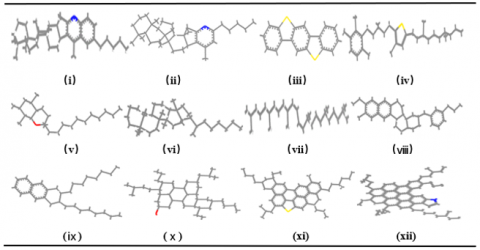Figure 1. A diagram of the 4-component 12-molecule structure

## 2.png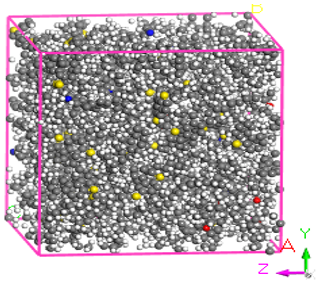Figure 2. Model of basic asphalt

## 3.png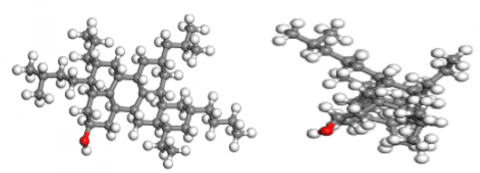Figure 3. Comparison of molecule before and after optimization: (a) Colloidal molecule before optimization; (b) Colloidal molecule after optimization

2.2 Verification of the asphalt model

RDF can describe the orderly distribution of asphalt molecules in space. Within a certain distance, the distribution is orderly, after exceeding the distance, the distribution becomes disorderly.

$g(r)=\frac{1}{4 \rho \pi r^{2}} \frac{\sum_{i=1}^{r} \sum_{j=1}^{n} \Delta N(r \rightarrow r+\xi r)}{N \times T}$    (1)

where , ρ represents the system density (Kg/cm3); r represents the distance between ions (m); N represents the total number of particles; ΔN represents the number of molecules in the system interval; T represents the system calculation time (ps); ξ is an infinitesimal constant.

## 4.png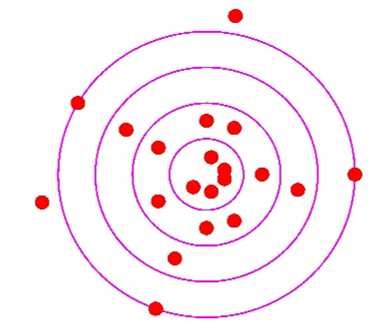Figure 4. A diagram of the concept of the RDF of particles

## 5.png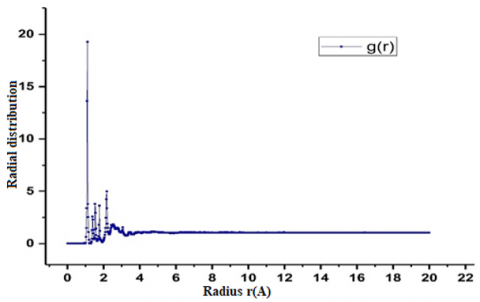Figure 5. Radial distribution of basic asphalt particles

Figure 4 above shows that an asphalt molecule in the system is located at the center of a space sphere with a radius of r, and the atoms beyond the range of r exhibit a disordered state. Figure 5 gives a diagram of the RDF of basic asphalt system, in the figure, atoms gather at the position of r=1Å (1Å=0.1nm); within the range with a radius of 1-5Å, the atoms in the asphalt model distribute orderly; when the distance exceeds 5Å, g(r) becomes very smooth and gradually approaches 1, indicating that the spatial arrangement of the asphalt model shows disordered distribution at the far end, which is consistent with the actual spatial distribution of asphalt particles.

2.2.2 Solubility

The solubility is the degree of compatibility of multiple substances with each other after they are mixed. Wang et al.  used the solubility parameter to evaluate the compatibility of SBS modifier and asphalt material. Solubility is the degree of mixing of molecules in the mixture and the interaction of the functional groups, therefore it can be determined by the average energy. Cohesive energy density (CED) is the energy required for 1 mol of polymers per unit volume to overcome intermolecular forces and become gas, it can be calculated using the molecular simulation software and then the solubility parameter δ could be determined (such as Table 2):

$C E D=E_{\text {coh }} / V$    (2)

$\delta=\sqrt{C E D}$    (3)

where, Ecoh is the energy required for molecules in the system to diffuse to infinity; CED represents the cohesive energy density (J/cm3).

The density of the asphalt molecule model was calculated to be 0.99g/cm3, its deviation from the actual density value was within 5%, which met the requirement and the solubility was within the experimental data range of asphalt, and this proved that the proposed asphalt molecule model can represent real asphalt molecules.

Table 2. Asphalt model parameter verification

 Structural parameter Density(g/cm3) Solubility(J/cm3)1/2 Simulation result 0.99 18.26 Experiment result [14, 15] 1.02-1.04 13.30-22.50
3. Introduction of Modifiers

Rubber modifiers are widely used in road asphalt materials. They can improve the mechanical properties of asphalt and increase its viscosity; the rubber molecules are high molecular polymers formed by the polymerization of butadiene. The single chains of rubber molecules are optimized according to the steps of structural optimization of basic asphalt to obtain the stable structure. NR molecule has a cis structure, and the TPI molecule is an isomer of NR . The structure of TPI molecule is trans-1,4-polyisoprene, and its linear structure is shown in Figure 6.

## 6.png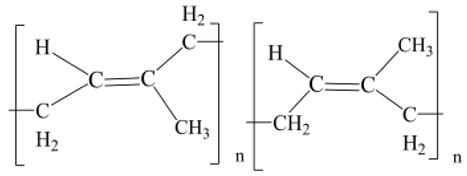Figure 6. Structures of TPI and NR

4. Self-Healing Model

4.1 Modeling

The basic asphalt, the TPI-modified asphalt, and the NR-modified asphalt were modeled respectively. First the models were geometrically optimized at 300K-500K in the minimum energy state, and then under NVT ensemble and NPT ensemble, they were subject to model molecular optimization for 150ps so that the model systems were optimized to the greatest extent. For the stable systems of basic asphalt and modified asphalt, the Build Layers task in the Build module was used to build self-healing models, and a 10Å vacuum layer was used to represent the width of the self-healing microcrack. The 150ps NPT ensemble in MD of the Forcite module was run to simulate the self-healing phenomenon of asphalt, the COMPASSⅡ force field was adopted, and the temperature was set to 303.15K, 313.15K, 323.15K, 333.15K, and 343.15K (303.15K=30℃) respectively, the pressure was 0.0001Gpa standard atmospheric pressure, the algorithm was optimized for 1,500,000 steps, the time step was 0.1fs, and each structure was optimized for 5,000 steps. For the initial model systems, there’re OA=37.58Å, OB=37.58Å, OC=117.55Å (In Figure 7 C: OA is the width of the model system, OB is the height, and OC is the length). In terms of the density data of the self-healing process, the initial density of the structure system was 0.6170g/cm3, the system had a 10Å vacuum layer, in molecular dynamics, the volume of the system became smaller, the length of each edge (OA, OB, OC) was shortened, the molecules moved to the vacuum layer, and finally reached an equilibrium state, the density was 0.99g/cm3, which was consistent with the actual healing situation.

## 7.png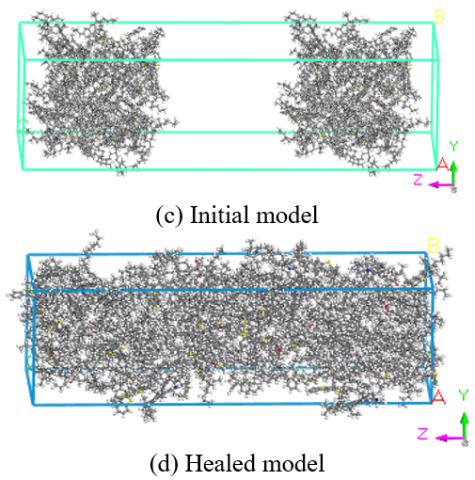Figure 7. Models of basic asphalt (10Å) before and after healing

4.2 Process of the healing model

(1) Between 0-50ps, the asphalt self-healing system is in a turbulent stage at the beginning, the density curve decreases rapidly and then increases fast. In Figure 8, during the dynamic molecule movement, the asphalt molecules adjust their own temperature and convert the energy they have into kinetic energy to make the molecules be able to move; under the NPT ensemble, the density curve increases fast after the fluctuation period, and the molecules can move stably and rapidly, the density curve shows that the change of density is approximately proportional to the movement time.

(2) Between 50-100ps, the density curve changes slowly. In the self-healing model, molecules diffuse to the two ends of the vacuum layer. In the process of dynamic optimization of the system, the volume of the model is gradually compressed, and the density shows an upward trend. Asphalt molecules move stably, and the healing model performs structure restoration.

(3) Between 100-150ps, the healing density curve grows slowly, the molecules are well distributed in the system. In the healing model, the vacuum layer and the porous sections at both ends are distributed by the diffused molecules. In Figure 9, the density curve fluctuates slightly and stabilizes at a certain value, the intermolecular structure and performance of the asphalt system return to the initial state.

## 8.png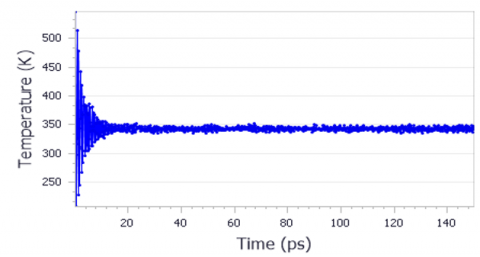Figure 8. Temperature distribution of the basic asphalt -10Å model

## 9.png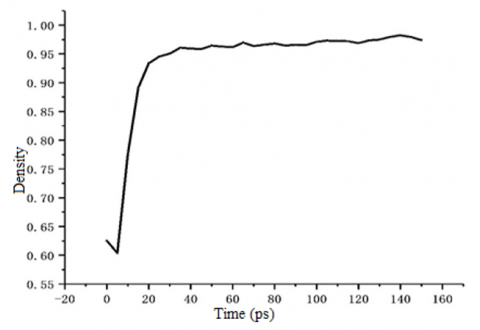Figure 9. Density curve of 10Å asphalt system

4.3 Energy change of the model system

Figure 10 shows the energy change of the basic asphalt -10Å system running for 150ps in the NPT ensemble, as can be seen in the figure, between 0-20ps, the potential energy and kinetic energy of the model are in a dynamic fluctuated state, the non-bond energy of the structure reduces to a certain value, the energy of asphalt molecules is given to the structure via temperature, in the healing system, the molecules move and distribute in the vacuum layer to restore the density and the structure; between 20-150ps, the structural energy is a balanced fluctuated value, indicating that the molecules in the system are in dynamic motion, and they restore the performance of the system.

According to Figure 11, in the basic asphalt -10Å system, the spatial angle is set to 90 degrees, the structure is a rectangular shaped structure and remains unchanged during the model running period, the OA, OB, and OC of the system change, and the changes in the two directions of OA and OB coincide, which is the same with the change trend in the OC direction. Between 0-20ps, the changes of the three directions stretch to the greatest extent first and reduce to the dynamic equilibrium value latter, which are consistent with the change trend of density in Figure 10 (fast decreasing first and fast increasing latter), and this indicates that the structure fluctuates in the system due to the existence of energy molecules, which gives the asphalt molecules energy to diffuse in and heal the system; between 20-150ps, the asphalt healing model is in a dynamic distribution state, the system heals stably and restores to the initial state performance.

## 10.png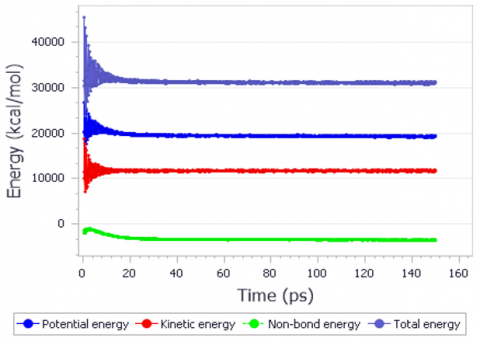Figure 10. Energy of basic asphalt -10Å system

## 11.pngFigure 11. Cell length and angle of basic asphalt -10Å system

4.4 Diffusion coefficient

According to the theory proposed by Einstein that the average of the sum of the squares of the random moving distances of particles is proportional to time, the derived diffusion coefficient formula is:

$<r^{2}>=6 D t+C$    (4)

where, <r2> is the average displacement; D is the diffusion coefficient; C is a constant; MSD is the mean square displacement of the model system, it represents the square of the distance between the position of a random particle in the asphalt molecule at time t and the initial position of the particle.

$M S D_{(\Delta t)}=<[r(t-\Delta t)-r(t)]^{2}>$    (5)

Then the formula of the diffusion coefficient is:

$D=\frac{1}{6 t} \lim _{t \rightarrow \infty} \frac{d M S D}{d \Delta t}$    (6)

In the dynamic simulation of self-healing models, the MSD analysis task in the Analysis of the Forceite module was adopted for each model and it was set to calculate the structure models every 5ps, the self-healing models of 1500000 simulation steps were calculated, and the MSD curves of each model are shown in Figure 11, according to the curves, we can know the entire MSD curves of the 10Å self-healing models of the NR and TPI systems under different temperatures of 303.15K, 313.15K, 323.15K, 333.15K, and 343.15K.

## 12.png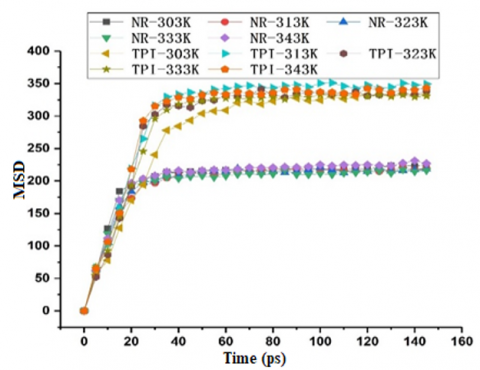Figure 12. MSD curves of 10Å self-healing models under different temperatures

As shown in the Figure 12 above, MSD curves have three different slopes, respectively from 0 to 40ps, from 40 to 80ps, and from 80 to 150ps. According to the curves of asphalt healing density, before 40ps is the asphalt self-healing process, at this stage, the diffusion of the asphalt self-healing model is mainly the recovery of strength and structure. After 40ps, the structure restoration is gradually completed, at this stage, the diffusion is mainly the restoration of the properties of the asphalt, its properties restore and close to the original state. After 80ps is the asphalt self-diffusion stage after the healing is completed. The 0-40ps stage is the most representative stage and is selected for the calculation of diffusion coefficient, this stage is the longest one and a part of the asphalt healing process, and it is conductive to understanding the change of the diffusion coefficient of asphalt molecules during the asphalt healing process. Under the five temperature conditions, the healing curves of TPI models were above those of NR models, and the MSD values of TPI were greater than those of the NR models, indicating that TPI’ diffusion performance is better than NR, but for the NR-modified asphalt, when MSD was 20ps, the curves reached and fluctuated up and down around a stable value, and it reached the equilibrium value before TPI, adding rubber modifiers in the healing models has little impact on temperature. The diffusion coefficients of each model calculated by formulas (4), (5), and (6) are shown in Table 3 (unit: (m-2×s-1)-1):

Table 3. Diffusion coefficients of each self-healing model (×10-8)

 Temperature (K) 303.15 313.15 323.15 333.15 343.15 TPI 1.3 1.32 1.46 1.6 1.64 NR 1.41 1.51 1.55 1.66 1.67

Table 3 lists the diffusion coefficient values of rubber-modified asphalt under five temperature conditions. The values of the diffusion coefficient of the two types of modified asphalt increased with the temperature increment, indicating that rubber-modified asphalt has temperature sensitivity; under a same temperature, the diffusion coefficient of NR-modified asphalt is greater than that of TPI-modified asphalt, and its movement performance is better. The MSD curves of TPI-modified asphalt are above those of NR-modified asphalt, and the values are about 1.5 times. The viscosity of TPI-modified asphalt is greater than that of NR-modified asphalt, the movement of the modified asphalt molecules promoted the healing of the microcracks in the models.

5. Conclusions

(1) Through three parameters of density, solubility, and MSD, the representativeness and validity of the basic asphalt model had been verified.

(2) A few self-healing models were constructed to simulate the microcracks and the healing process of the asphalt was simulated in the NPT ensemble, the healing process of TPI-modified asphalt and NR-modified asphalt was analyzed; the balance points of MSD curves of NR-modified asphalt appeared before those of TPI-modified asphalt, indicating that the movement of NR-modified asphalt is better, and it can enhance the self-healing performance of the asphalt.

(3) As the temperature rises, the self-healing performance of rubber-modified asphalt is improved; under a same temperature, the self-healing performance improvement effect of NR is better than that of TPI.

References

 Zhu, F., Yue, H., Zu, E.F. (2005). Research and application of new functional material gutta percha. Journal of Anhui University Natural Science Edition, 29(3): 89-94.

 Xu, C., Yin, M.Z., Gao, W.J. (2012). Molecular dynamics simulation on mechanical properties of TPI/NR blends. China Adhesives, 21(7): 1-3.

 Zhang, H.J., Yue, H., Zhuang, C.Q., Liu, Q. (2011). Molecular Dynamics Simulation on the Glass Transition Temperatures of Tpi/Nr/Ldpe. Plastics, 40(1): 100-102, 127.

 Wang, W.Y. (2018). Design and synthesis of self-healing elastomer materials based on eucommic ulmoides gum and natural rubber. Shenyang University of Chemical Technology.

 Xuan, W.A., Xiong, J.P., Wei, W.F. (2020). Self-healing properties study of rubber asphalt based on viscoelastic theory. Highway, 65(1): 195-200.

 Rogel, E., Carbognani, L. (2003). Density estimation of asphaltenes using molecular dynamics simulations. Energy & Fuels, 17(2): 378-386. https://doi.org/10.1021/ef020200r

 Su, M.M., Zhang, H.L., Zhang, Y.P., Zhang, Z.P. (2017). Miscibility and mechanical properties of SBS and asphalt blends based on molecular dynamics simulation. Journal of Chang'an University (Natural Science Edition), 37(3): 24-32. https://doi.org/10.3969/j.issn.1671-8879.2017.03.004

 Zhang, L., Greenfield, M.L. (2007). Analyzing properties of model asphalts using molecular simulation. Energy & Fuels, 21(3): 1712-1716. https://doi.org/10.1021/ef060658j

 Zhang, L., Greenfield, M.L. (2008). Effects of polymer modification on properties and microstructure of model asphalt systems. Energy & Fuels, 22(5): 3363-3375. https://doi.org/10.1021/ef700699p

 Li, D.D., Greenfield, M.L. (2011). High internal energies of proposed asphaltene structures. Energy & Fuels, 25(8): 3698-3705. https://doi.org/10.1021/ef200507c

 Li, D.D., Greenfield, M.L. (2014). Chemical compositions of improved model asphalt systems for molecular simulations. Fuel, 115: 347-356. https://doi.org/10.1016/j.fuel.2013.07.012

 Wu, X.R. (2010). Synthesis and characterization analysis of poss hybrid nanocomposite. Harbin Institute of Technology.

 Wang, S.J., Zheng, Y.C., Ding, Y.J. (2013). Research on compatibility of monomer grafted SBS and asphalt based on molecular simulation technology. Highways & Automotive Applications, 2013(1): 100-102. https://doi.org/10.3969/j.issn.1671-2668.2013.01.027

 Robertson, R.E., Branthaver, J.F., Harnsberger, P.M., Petersen, J.C., Dorrence, S.M., McKay, J.F., Turner, T.F., Pauli, A.T., Huang, S.C. (2001). Fundamental Properties of Asphalt and Modifified Asphalts, Volume I: Interpretive Report. See also FHWA-RD-99-213, Volume II: Final Report, New Methods.

 Painter, P.C. (1993). The characterization of asphalt and asphalt recyclability. Strategic Highway Research Program, National Research Council, 675: 1-5.

 Yan, R.F. (1991). New Progress in Research on Eucommia Gum Chemical Bulletin, 1991(1): 1-6. https://doi.org/10.14159/j.cnki.0441-3776.1991.01.001